# Plotting different types of plots using Factor plot in seaborn

Prerequisites: Introduction to Seaborn

Seaborn is an amazing visualization library for statistical graphics plotting in Python. It provides beautiful default styles and color palettes to make statistical plots more attractive. It is built on the top of matplotlib library and also closely integrated to the data structures from pandas.

## Factor Plot

`Factor Plot` is used to draw a different types of `categorical plot`. The default plot that is shown is a point plot, but we can plot other seaborn categorical plots by using of `kind` parameter, like box plots, violin plots, bar plots, or strip plots.

Dataset Snippet :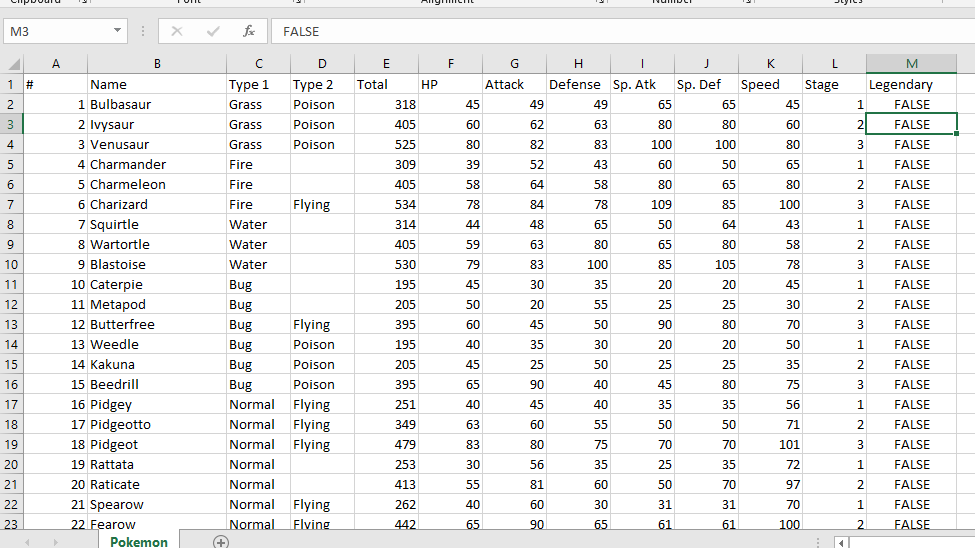Code 1: Point plot using factorplot() method of seaborn.

 `# importing required library ` `import` `pandas as pd ` `import` `seaborn as sns ` `import` `matplotlib.pyplot as plt ` ` `  `# read a csv file ` `df ``=` `pd.read_csv(``'Pokemon.csv'``) ` ` `  `# Stage v / s Attack point plot  ` `sns.factorplot(x ``=``'Stage'``, y ``=``'Attack'``, data ``=` `df) ` `sns.factorplot(x ``=``'Stage'``, y ``=``'Defense'``, data ``=` `df) ` ` `  `# Show the plots ` `plt.show() `

Output: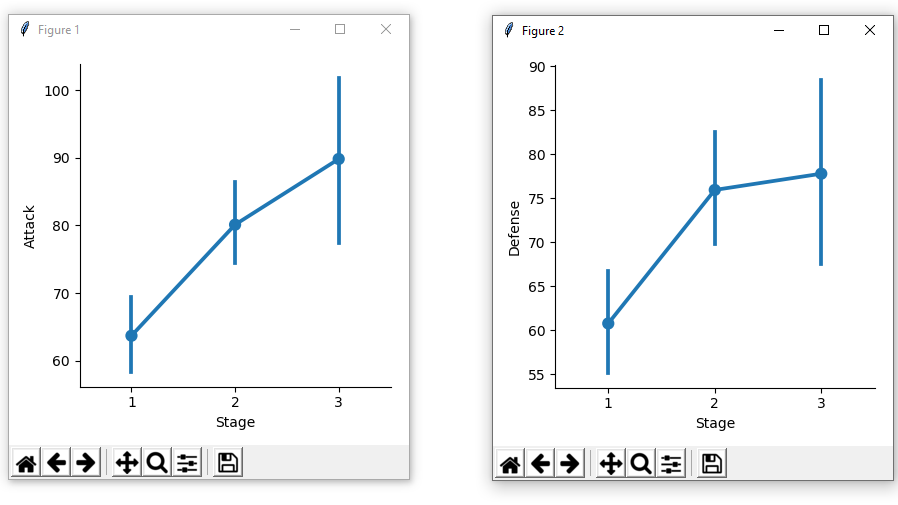Code 2: Violin plot using factorplot() method of seaborn.

 `# importing required library ` `import` `pandas as pd ` `import` `seaborn as sns ` `import` `matplotlib.pyplot as plt ` ` `  `# read a csv file ` `df ``=` `pd.read_csv(``'Pokemon.csv'``) ` ` `  `# Type 1 v / s Attack violin plot  ` `sns.factorplot(x ``=``'Type 1'``, y ``=``'Attack'``, ` `               ``kind ``=` `'violin'``, data ``=` `df) ` ` `  `# show the plots ` `plt.show() `

Output: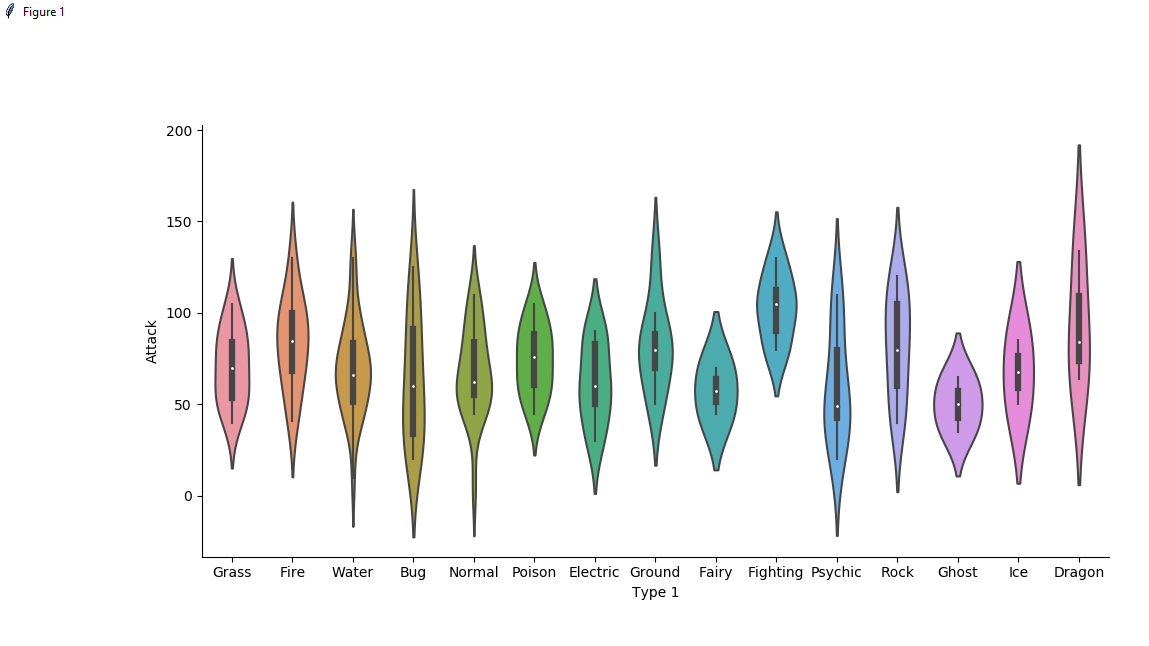Code 3: Bar plot using factorplot() method of seaborn.

 `# importing required library ` `import` `pandas as pd ` `import` `seaborn as sns ` `import` `matplotlib.pyplot as plt ` ` `  `# read a csv file ` `df ``=` `pd.read_csv(``'Pokemon.csv'``) ` ` `  `# Type 1 v / s Defense bar plot  ` `# with Stage column is used for  ` `# colour encoding i.e  ` `# on the basis of Stages different ` `# colours is decided, here in this ` `# dataset, 3 Stage is mention so  ` `# 3 different colours is used. ` `sns.factorplot(x ``=``'Type 1'``, y ``=``'Defense'``,  ` `               ``kind ``=` `'bar'``, hue ``=` `'Stage'``,  ` `               ``data ``=` `df) ` ` `  `# show the plots ` `plt.show() `

Output: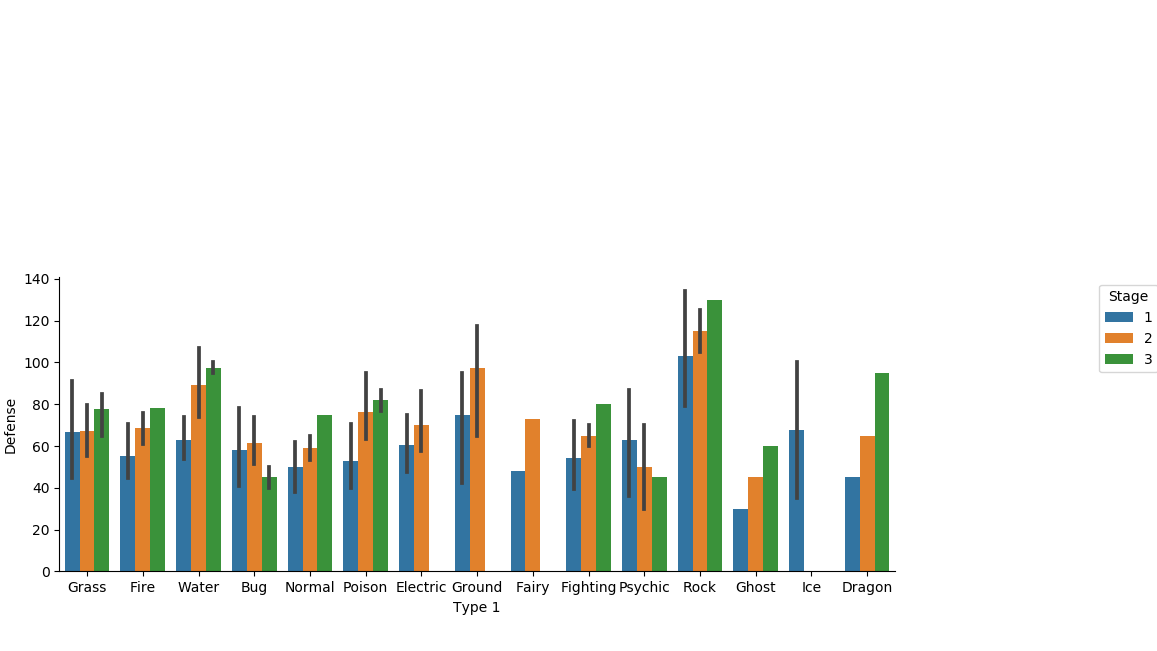Code 4: Box plot using factorplot() method of seaborn.

 `# importing required library ` `import` `pandas as pd ` `import` `seaborn as sns ` `import` `matplotlib.pyplot as plt ` ` `  `# read a csv file ` `df ``=` `pd.read_csv(``'Pokemon.csv'``) ` ` `  `# Stage v / s Defense box plot  ` `sns.factorplot(x ``=``'Stage'``, y ``=``'Defense'``, ` `               ``kind ``=` `'box'``, data ``=` `df) ` ` `  `# show the plots ` `plt.show() `

Output: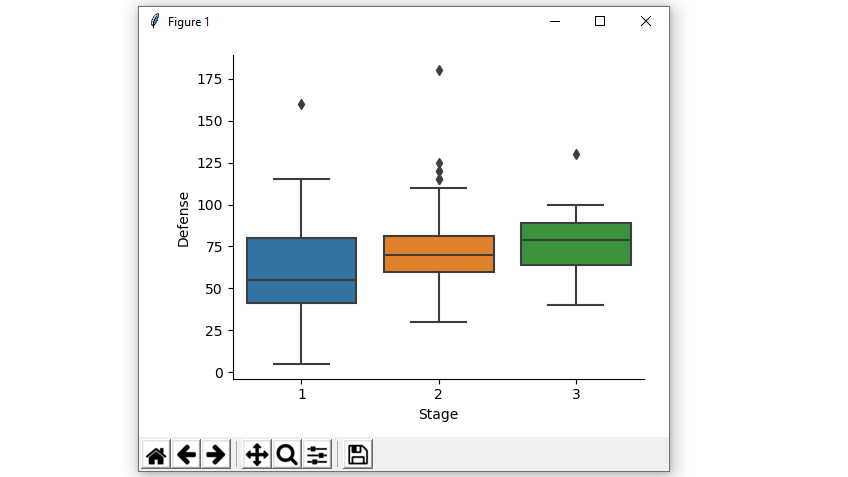Code 5: Strip plot using factorplot() method of seaborn.

 `# importing required library ` `import` `pandas as pd ` `import` `seaborn as sns ` `import` `matplotlib.pyplot as plt ` ` `  `# read a csv file ` `df ``=` `pd.read_csv(``'Pokemon.csv'``) ` ` `  `# Stage v / s Defense strip plot  ` `sns.factorplot(x ``=``'Stage'``, y ``=``'Defense'``,  ` `               ``kind ``=` `'strip'``, data ``=` `df) ` ` `  `# show the plots ` `plt.show() `

Output: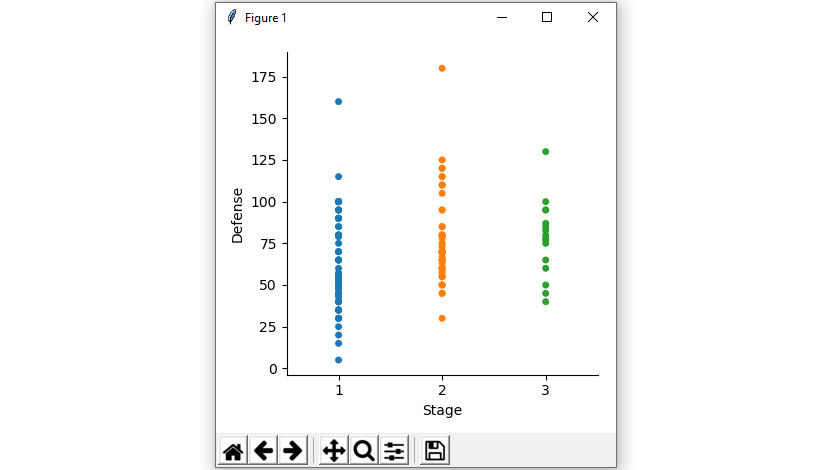Code 6: Count plot using factorplot() method of seaborn.

 `# importing required library ` `import` `pandas as pd ` `import` `seaborn as sns ` `import` `matplotlib.pyplot as plt ` ` `  `# read a csv file ` `df ``=` `pd.read_csv(``'Pokemon.csv'``) ` ` `  `# Stage v / s count - count plot  ` `sns.factorplot(x ``=``'Stage'``, kind ``=` `'count'``, data ``=` `df) ` ` `  `# show the plots ` `plt.show() `

Output: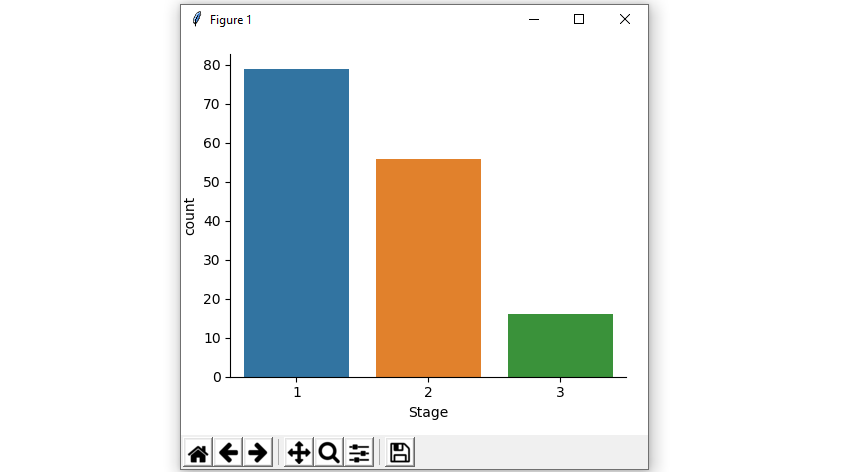Whether you're preparing for your first job interview or aiming to upskill in this ever-evolving tech landscape, GeeksforGeeks Courses are your key to success. We provide top-quality content at affordable prices, all geared towards accelerating your growth in a time-bound manner. Join the millions we've already empowered, and we're here to do the same for you. Don't miss out - check it out now!